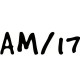Class 10 Maths Notes

# CBSE Class 10 Maths Chapter 13 Surface Areas VolumesWritten by
Surface Areas VolumesSurface Areas Volumes

### Surface Area Volumes

We will study Surface Area of a Combination of Solids, Volume of a Combination of Solids, Conversion of Solid from One Shape to Another & Frustum of a Cone .
Some of the basic solids are Cylinder, Cuboid, Sphere and Cone. Surface Area & volumes of these basic solids can easily be calculated using simple formulas.In our day-to-day life, we come across a number of solids made up of combinations of two or more of the basic solids such as cylinder, cone, sphere & cuboid. We can find volumes & surface area of these figures by breaking them into basic solids.

### Surface Area of Combination of Solids

As seen, we can easily break a complex solid into basic solids.
Total surface area of the complex solid is the sum of the curved surface areas of each of the individual basic parts
Numerical:  A vessel is in the form of a hollow hemisphere mounted by a hollow cylinder. The diameter of the hemisphere is 14 cm and the total height of the vessel is 13 cm. Find the inner surface area of the vessel.Solution: We can break this vessel into a cylinder & Hemisphere as then find the surface area.
Total inner Surface Area of Vessel = Inner surface area of (Cylinder A + Hemisphere B)
= 2πrh + 2πr
= 2 * 22/7 * 7 * 6 + 2 * 22/7 * 7 * 7  cm2
= 572 cm2

### Volume of Combination of Solids

As seen, we can easily break a complex solid into basic solids.
Volume of the complex solid is the sum of the volume of each of the individual basic parts. It may be noted that in calculating the surface area, we have not added the surface areas of the two constituents, because some part of the surface area disappeared in the process of joining them. However, this will not be the case when we calculate the volume. The volume of the solid formed by joining two basic solids will actually be the sum of the volumes of the constituents.Numerical: Find volume of  a spherical glass vessel has a cylindrical neck 8 cm long, 2 cm in diameter; diameter Of spherical part is 8.5 cm.
Volume of Object = Volume of Sphere + Volume of Cylinder
= 4/3 πr3 + πr2h
= 4/3 * 22/7 * (8.5)3 + 22/7 * (1)2 * 8  cm3
= 346.5 cm3

### Conversion of Solid from One Shape to Another

A Solid object e.g. wax, Iron etc is often heated to make it liquid & then cooled in a container with different shape to change the shape of solid. In this process, the shape is changed, but the volume remains same.
Numerical: How many silver coins, 1.75 cm in diameter and of thickness 2 mm, must be melted to form a cuboid of dimensions 5.5 cm × 10 cm × 3.5 cm?
Solution:  let’s assume x number of silver coins are melted to form the Cuboid, then volume of x coins will be equal to volume of Cuboid.
Coin is cylindrical in shape with radius 1.75/2 cm & height 2/10 cm.
Volume of 1 silver coin (Cylinder) is πr2h
Volume of x silver coins (Cylinder) is xπr2h   = x * 22/7 * 1.75 * 1.75 * 2/10  cm3    —-(i)
Volume of Cuboid = length * breadth * height = 5.5 * 10 * 3.5 cm3                  —-(ii)
Equating equation I & ii, that is volume of Cuboid = volume of x coins
Or    x * 22/7 * 1.75 * 1.75 * 2/10  cm3      =  5.5 * 10 * 3.5 cm3
or x =400
Thus 400 coins we melted.

### Frustum of a Cone

We will take a right circular cone and remove a portion of it. There are so many ways in which we can do this. But one particular case that we are interested in is the removal of a smaller right circular cone by cutting the given cone by a plane parallel to its base. You must have observed that the glasses, used for drinking water, are of this shape.  This remaining portion of cone is called Frustum of a cone.Let h be the height, l the slant height and r1 and r2 the radii of the ends (r1 > r2) of the frustum of a cone. Then we can directly find the volume, the curved surace area and the total surface area of frustum by using the formulae given below.Numerical: The cap used by the Turks, is shaped like the frustum of a cone.  If its radius on the open side is 10 cm, radius at the upper base is 4 cm and its slant height is 15 cm, find area of material used for making it.

Solution: Surface area of the cap is π(r1 + r2)l
Total Surface Area of cap (hollow from bottom)  = π(r1 + r2)l  + π( r2)2

= 22/7 *( 10 + 4 ) * 15 + 22/7 * 4 * 4 cm2
= 710.3 cm2

### MATHS Revision Notes

Chapter:01  Real Numbers System
Chapter:02  Polynomials
Chapter:03  Pair of Linear Equations in Two Variables
Chapter:05  Arithemetic Progressions
Chapter:06  Triangles
Chapter:07  Coordinate Geometry
Chapter:08  Introduction to Trignometry
Chapter:09  Some Application Of Trignometry
Chapter:10  Circles
Chapter:11  Constructions
Chapter:12  Area Related to Cirles
Chapter:13  Surface Area Volume
Chapter:14  Stastistics
Chapter:15  Probability

Science Revision Notes

English Revision Notes

Economics Revision Notes